# How to use polygons or lines as exposure#

## Introduction#

Exposure in CLIMADA are usually represented as individual points or a raster of points. See Exposures tutorial to learn how to fill and use exposures. In this tutorial we show you how to run the impact calculation chain if you have polygons or lines to start with. The approach provides an all-in-one method for impact calculation: calc_geom_impact It features three sub-steps, for which the current util module lines_polys_handler also provides separate functions:

1. Disaggregation of line and polygon data into point exposure:

• Interpolate geometries to points to fit in an Exposure instance;

• Disaggregate the respective geometry values to the point values

2. Perform the impact calculation in CLIMADA with the point exposure

3. Aggregate the calculated point Impact back to an impact instance for the initial polygons or lines

## Quick example#

Get example polygons (provinces), lines (rails), points exposure for the Netherlands, and create one single Exposures. Get demo winter storm hazard and a corresponding impact function.

from climada.util.api_client import Client

HAZ = Client().get_hazard('storm_europe', name='test_haz_WS_nl', status='test_dataset');

EXP_POLY = Client().get_exposures('base', name='test_polygon_exp', status='test_dataset');
EXP_LINE = Client().get_exposures('base', name='test_line_exp', status='test_dataset');
EXP_POINT = Client().get_exposures('base', name='test_point_exp', status='test_dataset');

EXP_MIX = Exposures.concat([EXP_POLY, EXP_LINE, EXP_POINT])

IMPF = ImpfStormEurope.from_welker()
IMPF_SET = ImpactFuncSet([IMPF])


Compute the impact in one line.

#disaggregate in the same CRS as the exposures are defined (here degrees), resolution 1degree
#divide values on points
#aggregate by summing

impact = u_lp.calc_geom_impact(
exp=EXP_MIX, impf_set=IMPF_SET, haz=HAZ,
res=0.2, to_meters=False, disagg_met=u_lp.DisaggMethod.DIV, disagg_val=None,
agg_met=u_lp.AggMethod.SUM
)

2022-06-24 13:16:15,277 - climada.entity.exposures.base - INFO - Setting latitude and longitude attributes.
2022-06-24 13:16:15,284 - climada.entity.exposures.base - INFO - Matching 183 exposures with 9944 centroids.
2022-06-24 13:16:15,285 - climada.util.coordinates - INFO - No exact centroid match found. Reprojecting coordinates to nearest neighbor closer than the threshold = 100
2022-06-24 13:16:15,295 - climada.engine.impact - INFO - Exposures matching centroids found in centr_WS
2022-06-24 13:16:15,296 - climada.engine.impact - INFO - Calculating damage for 182 assets (>0) and 2 events.

/Users/ckropf/Documents/Climada/climada_python/climada/util/lines_polys_handler.py:931: UserWarning: Geometry is in a geographic CRS. Results from 'length' are likely incorrect. Use 'GeoSeries.to_crs()' to re-project geometries to a projected CRS before this operation.

line_lengths = gdf_lines.length

u_lp.plot_eai_exp_geom(impact);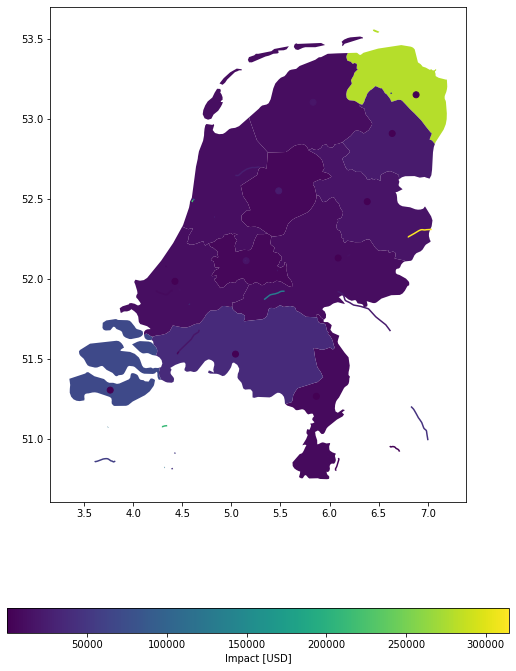#disaggregate in meters
#same value for each point, fixed to 1 (allows to get percentages of affected surface/distance)
#aggregate by summing

impact = u_lp.calc_geom_impact(
exp=EXP_MIX, impf_set=IMPF_SET, haz=HAZ,
res=1000, to_meters=True, disagg_met=u_lp.DisaggMethod.FIX, disagg_val=1.0,
agg_met=u_lp.AggMethod.SUM
);

2022-06-24 13:16:17,387 - climada.entity.exposures.base - INFO - Setting latitude and longitude attributes.
2022-06-24 13:16:18,069 - climada.entity.exposures.base - INFO - Matching 37357 exposures with 9944 centroids.
2022-06-24 13:16:18,073 - climada.util.coordinates - INFO - No exact centroid match found. Reprojecting coordinates to nearest neighbor closer than the threshold = 100
2022-06-24 13:16:18,114 - climada.engine.impact - INFO - Exposures matching centroids found in centr_WS
2022-06-24 13:16:18,117 - climada.engine.impact - INFO - Calculating damage for 37357 assets (>0) and 2 events.

import matplotlib.pyplot as plt
ax = u_lp.plot_eai_exp_geom(impact, legend_kwds={'label': 'percentage', 'orientation': 'horizontal'})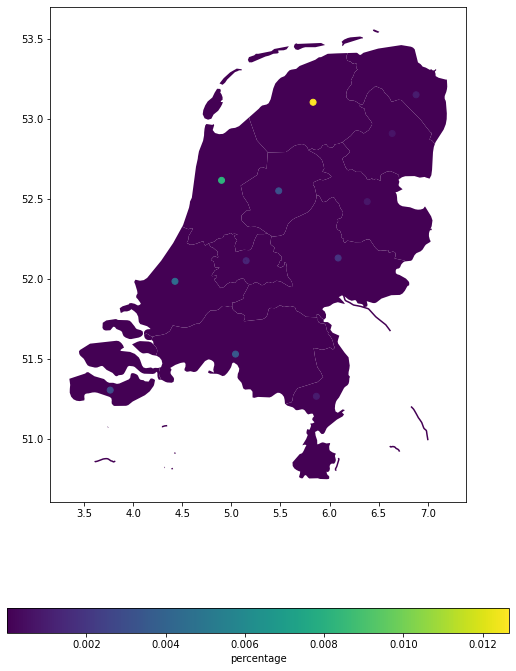## Polygons#

Polygons or shapes are a common geographical representation of countries, states etc. as for example in NaturalEarth. Map data, as for example buildings, etc. obtained from openstreetmap (see tutorial here), also frequently come as (multi-)polygons. Here we want to show you how to deal with exposure information as polygons.

Lets assume we have the following data given. The polygons of the admin-1 regions of the Netherlands and an exposure value each, which we gather in a geodataframe. We want to know the Impact of Lothar on each admin-1 region.

In this tutorial, we shall see how to compute impacts for exposures defined on shapely geometries (polygons and/or lines). The basic principle is to disaggregate the geometries to a raster of points, compute the impact per points, and then re-aggregate. To do so, several methods are available. Here is a brief overview.

# Imports
import geopandas as gpd
import pandas as pd
from pathlib import Path


def gdf_poly():

# open the file containing the Netherlands admin-1 polygons
category='cultural',

# extract the NL polygons
prov_names = {'Netherlands': ['Groningen', 'Drenthe',
'Overijssel', 'Gelderland',
'Limburg', 'Zeeland',
'Noord-Brabant', 'Zuid-Holland',
'Noord-Holland', 'Friesland',
'Flevoland', 'Utrecht']
}
polygon_Netherlands, polygons_prov_NL = country_iso_geom(prov_names,
shp_file)
prov_geom_NL = {prov: geom for prov, geom in zip(list(prov_names.values()), list(polygons_prov_NL.values()))}

# assign a value to each admin-1 area (assumption 100'000 USD per inhabitant)
population_prov_NL = {'Drenthe':493449, 'Flevoland':422202,
'Friesland':649988, 'Gelderland':2084478,
'Groningen':585881, 'Limburg':1118223,
'Noord-Brabant':2562566, 'Noord-Holland':2877909,
'Overijssel':1162215, 'Zuid-Holland':3705625,
'Utrecht':1353596, 'Zeeland':383689}
value_prov_NL = {n: 100000 * population_prov_NL[n] for n in population_prov_NL.keys()}

# combine into GeoDataFrame and add a coordinate reference system to it:
df1 = pd.DataFrame.from_dict(population_prov_NL, orient='index', columns=['population']).join(
pd.DataFrame.from_dict(value_prov_NL, orient='index', columns=['value']))
df1['geometry'] = [prov_geom_NL[prov] for prov in df1.index]
gdf_polys = gpd.GeoDataFrame(df1)
gdf_polys = gdf_polys.set_crs(epsg=4326)
return gdf_polys

exp_nl_poly = Exposures(gdf_poly())
exp_nl_poly.gdf['impf_WS'] = 1

population value geometry impf_WS
Drenthe 493449 49344900000 POLYGON ((7.07215 52.84132, 7.06198 52.82401, ... 1
Flevoland 422202 42220200000 POLYGON ((5.74046 52.83874, 5.75012 52.83507, ... 1
Friesland 649988 64998800000 MULTIPOLYGON (((5.17977 53.00899, 5.27947 53.0... 1
Gelderland 2084478 208447800000 POLYGON ((6.77158 52.10879, 6.76587 52.10840, ... 1
Groningen 585881 58588100000 MULTIPOLYGON (((7.19459 53.24502, 7.19747 53.2... 1
# take a look
exp_nl_poly.gdf.plot('value', legend=True, cmap='OrRd')

<AxesSubplot:>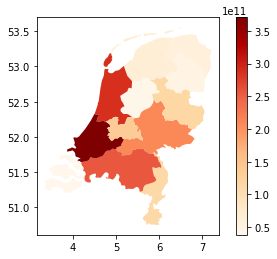# define hazard
storms = StormEurope.from_footprints(WS_DEMO_NC)
# define impact function
impf = ImpfStormEurope.from_welker()
impf_set = ImpactFuncSet([impf])

2022-06-24 13:16:20,039 - climada.hazard.storm_europe - INFO - Constructing centroids from /Users/ckropf/climada/demo/data/fp_lothar_crop-test.nc
2022-06-24 13:16:20,124 - climada.hazard.centroids.centr - INFO - Convert centroids to GeoSeries of Point shapes.

/Users/ckropf/Documents/Climada/climada_python/climada/hazard/centroids/centr.py:822: UserWarning: Geometry is in a geographic CRS. Results from 'buffer' are likely incorrect. Use 'GeoSeries.to_crs()' to re-project geometries to a projected CRS before this operation.

xy_pixels = self.geometry.buffer(res / 2).envelope

2022-06-24 13:16:22,004 - climada.hazard.storm_europe - INFO - Commencing to iterate over netCDF files.


### Compute polygon impacts - all in one#

All in one: The main method calc_geom_impact provides several disaggregation keywords, specifiying

• the target resolution (res),

• the method on how to distribute the values of the original geometries onto the newly generated interpolated points (disagg_met)

• the source (and number) of the value to be distributed (disagg_val).

• the aggregation method (agg_met)

disagg_met can be either fixed (FIX), replicating the original shape’s value onto all points, or divided evenly (DIV), in which case the value is divided equally onto all new points. disagg_val can either be taken directly from the exposure gdf’s value column (None) or be indicated here explicitly (float). Resolution can be given in the gdf’s original (mostly degrees lat/lon) format, or in metres. agg_met can currently be only (SUM) were the value is summed over all points in the geometry.

Polygons can also be disaggregated on a given fixed grid, see example below

Example 1: Target resolution in degrees lat/lon, equal (average) distribution of values from exposure gdf among points.

imp_deg = u_lp.calc_geom_impact(
exp=exp_nl_poly, impf_set=impf_set, haz=storms,
res=0.005, disagg_met=u_lp.DisaggMethod.DIV, disagg_val=None,
agg_met=u_lp.AggMethod.SUM
)

2022-06-24 13:16:30,535 - climada.entity.exposures.base - INFO - Setting latitude and longitude attributes.
2022-06-24 13:16:33,946 - climada.entity.exposures.base - INFO - Matching 195323 exposures with 9944 centroids.
2022-06-24 13:16:33,950 - climada.util.coordinates - INFO - No exact centroid match found. Reprojecting coordinates to nearest neighbor closer than the threshold = 100
2022-06-24 13:16:34,140 - climada.engine.impact - INFO - Exposures matching centroids found in centr_WS
2022-06-24 13:16:34,144 - climada.engine.impact - INFO - Calculating damage for 195323 assets (>0) and 2 events.

u_lp.plot_eai_exp_geom(imp_deg);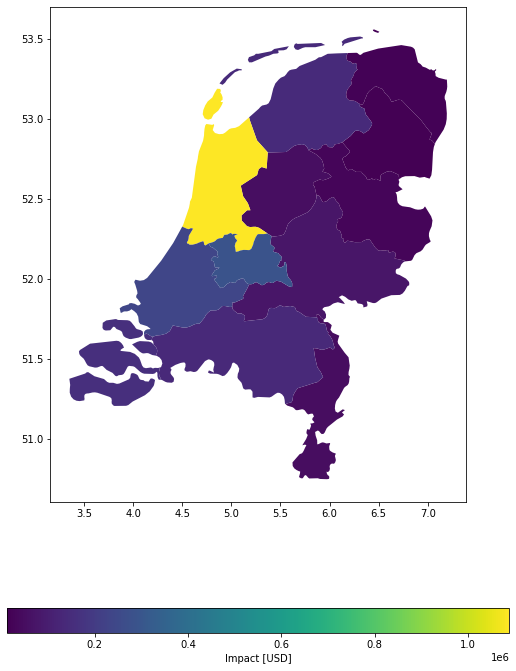Example 2: Target resolution in metres, equal (divide) distribution of values from exposure gdf among points.

imp_m = u_lp.calc_geom_impact(
exp=exp_nl_poly, impf_set=impf_set, haz=storms,
res=500, to_meters=True, disagg_met=u_lp.DisaggMethod.DIV, disagg_val=None,
agg_met=u_lp.AggMethod.SUM
)

2022-06-24 13:16:41,366 - climada.entity.exposures.base - INFO - Setting latitude and longitude attributes.
2022-06-24 13:16:44,164 - climada.entity.exposures.base - INFO - Matching 148369 exposures with 9944 centroids.
2022-06-24 13:16:44,168 - climada.util.coordinates - INFO - No exact centroid match found. Reprojecting coordinates to nearest neighbor closer than the threshold = 100
2022-06-24 13:16:44,387 - climada.engine.impact - INFO - Exposures matching centroids found in centr_WS
2022-06-24 13:16:44,390 - climada.engine.impact - INFO - Calculating damage for 148369 assets (>0) and 2 events.

u_lp.plot_eai_exp_geom(imp_m);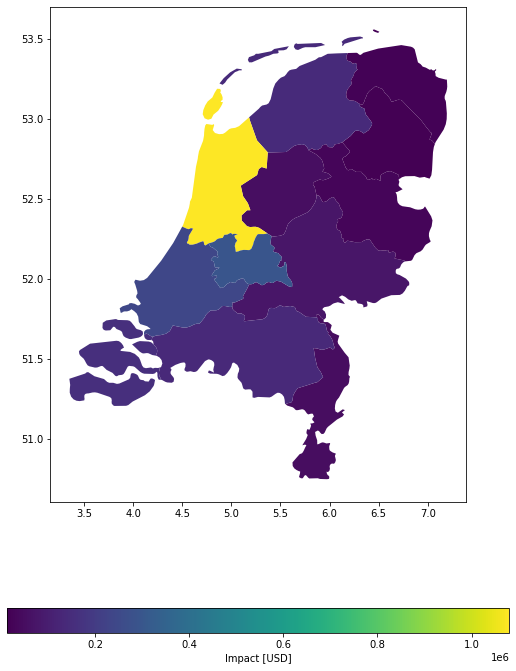For this specific case, both disaggregation methods provide a relatively similar result, given the chosen numbers:

(imp_deg.eai_exp - imp_m.eai_exp) / imp_deg.eai_exp

array([ 0.00614586, -0.01079377,  0.00021355,  0.00140608,  0.0038771 ,
-0.0066888 , -0.00171755,  0.00741871, -0.00107029,  0.00424221,
-0.01838225,  0.01811858])


Example 3: Target predefined grid, equal (divide) distribution of values from exposure gdf among points.

#regular grid from exposures bounds
res = 0.1
(_, _, xmax, ymax) = exp_nl_poly.gdf.geometry.bounds.max()
(xmin, ymin, _, _) = exp_nl_poly.gdf.geometry.bounds.min()
bounds = (xmin, ymin, xmax, ymax)
height, width, trafo = u_coord.pts_to_raster_meta(
bounds, (res, res)
)
x_grid, y_grid = u_coord.raster_to_meshgrid(trafo, width, height)

imp_g = u_lp.calc_grid_impact(
exp=exp_nl_poly, impf_set=impf_set, haz=storms,
grid=(x_grid, y_grid), disagg_met=u_lp.DisaggMethod.DIV, disagg_val=None,
agg_met=u_lp.AggMethod.SUM
)

2022-06-24 13:16:45,086 - climada.entity.exposures.base - INFO - Setting latitude and longitude attributes.
2022-06-24 13:16:45,100 - climada.entity.exposures.base - INFO - Matching 486 exposures with 9944 centroids.
2022-06-24 13:16:45,102 - climada.util.coordinates - INFO - No exact centroid match found. Reprojecting coordinates to nearest neighbor closer than the threshold = 100
2022-06-24 13:16:45,110 - climada.engine.impact - INFO - Exposures matching centroids found in centr_WS
2022-06-24 13:16:45,111 - climada.engine.impact - INFO - Calculating damage for 486 assets (>0) and 2 events.

u_lp.plot_eai_exp_geom(imp_g);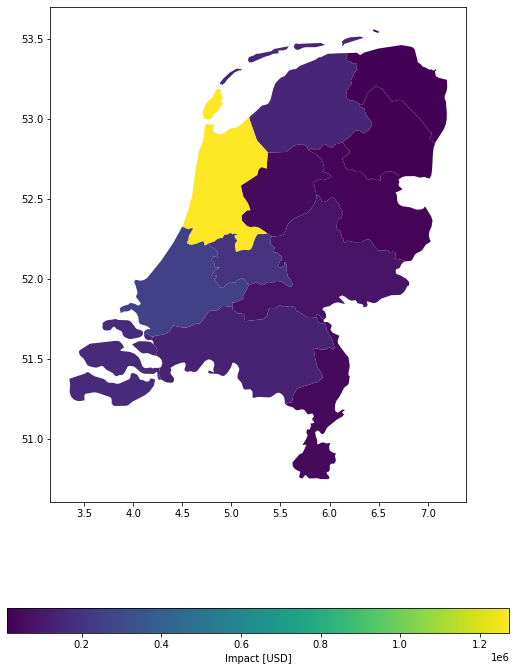### Compute polygon impacts - step by step#

Step 1: Disaggregate polygon exposures to points. It is useful to do this separately, when the discretized exposure is used several times, for example, to compute with different hazards.

Several disaggregation methods can be used as shown below:

# Disaggregate exposure to 10'000 metre grid, each point gets average value within polygon.
exp_pnt = u_lp.exp_geom_to_pnt(
exp_nl_poly, res=10000, to_meters=True,
disagg_met=u_lp.DisaggMethod.DIV, disagg_val=None
)

2022-06-24 13:16:45,445 - climada.entity.exposures.base - INFO - Setting latitude and longitude attributes.

population value geometry_orig impf_WS geometry latitude longitude
Drenthe 0 493449 2.056038e+09 POLYGON ((7.07215 52.84132, 7.06198 52.82401, ... 1 POINT (6.21931 52.75939) 52.759394 6.219308
1 493449 2.056038e+09 POLYGON ((7.07215 52.84132, 7.06198 52.82401, ... 1 POINT (6.30914 52.75939) 52.759394 6.309139
2 493449 2.056038e+09 POLYGON ((7.07215 52.84132, 7.06198 52.82401, ... 1 POINT (6.39897 52.75939) 52.759394 6.398971
3 493449 2.056038e+09 POLYGON ((7.07215 52.84132, 7.06198 52.82401, ... 1 POINT (6.48880 52.75939) 52.759394 6.488802
4 493449 2.056038e+09 POLYGON ((7.07215 52.84132, 7.06198 52.82401, ... 1 POINT (6.57863 52.75939) 52.759394 6.578634
# Disaggregate exposure to 0.1° grid, no value disaggregation specified --> replicate initial value
exp_pnt2 = u_lp.exp_geom_to_pnt(
exp_nl_poly, res=0.1, to_meters=False,
disagg_met=u_lp.DisaggMethod.FIX, disagg_val=None)

2022-06-24 13:16:45,516 - climada.entity.exposures.base - INFO - Setting latitude and longitude attributes.

population value geometry_orig impf_WS geometry latitude longitude
Drenthe 0 493449 49344900000 POLYGON ((7.07215 52.84132, 7.06198 52.82401, ... 1 POINT (6.22948 52.71147) 52.711466 6.229476
1 493449 49344900000 POLYGON ((7.07215 52.84132, 7.06198 52.82401, ... 1 POINT (6.32948 52.71147) 52.711466 6.329476
2 493449 49344900000 POLYGON ((7.07215 52.84132, 7.06198 52.82401, ... 1 POINT (6.42948 52.71147) 52.711466 6.429476
3 493449 49344900000 POLYGON ((7.07215 52.84132, 7.06198 52.82401, ... 1 POINT (6.52948 52.71147) 52.711466 6.529476
4 493449 49344900000 POLYGON ((7.07215 52.84132, 7.06198 52.82401, ... 1 POINT (6.62948 52.71147) 52.711466 6.629476
# Disaggregate exposure to 1'000 metre grid, each point gets value corresponding to
# its representative area (1'000^2).
exp_pnt3 = u_lp.exp_geom_to_pnt(
exp_nl_poly, res=1000, to_meters=True,
disagg_met=u_lp.DisaggMethod.FIX, disagg_val=10e6)

2022-06-24 13:16:47,258 - climada.entity.exposures.base - INFO - Setting latitude and longitude attributes.

population value geometry_orig impf_WS geometry latitude longitude
Drenthe 0 493449 10000000.0 POLYGON ((7.07215 52.84132, 7.06198 52.82401, ... 1 POINT (6.38100 52.62624) 52.626236 6.381005
1 493449 10000000.0 POLYGON ((7.07215 52.84132, 7.06198 52.82401, ... 1 POINT (6.38999 52.62624) 52.626236 6.389988
2 493449 10000000.0 POLYGON ((7.07215 52.84132, 7.06198 52.82401, ... 1 POINT (6.39897 52.62624) 52.626236 6.398971
3 493449 10000000.0 POLYGON ((7.07215 52.84132, 7.06198 52.82401, ... 1 POINT (6.40795 52.62624) 52.626236 6.407954
4 493449 10000000.0 POLYGON ((7.07215 52.84132, 7.06198 52.82401, ... 1 POINT (6.41694 52.62624) 52.626236 6.416937
# Disaggregate exposure to 1'000 metre grid, each point gets value corresponding to 1
# After dissagregation, each point has a value equal to the percentage of area of the polygon
exp_pnt4 = u_lp.exp_geom_to_pnt(
exp_nl_poly, res=1000, to_meters=True,
disagg_met=u_lp.DisaggMethod.DIV, disagg_val=1)
exp_pnt4.gdf.tail()

2022-06-24 13:16:49,929 - climada.entity.exposures.base - INFO - Setting latitude and longitude attributes.

population value geometry_orig impf_WS geometry latitude longitude
Zeeland 1897 383689 0.000526 MULTIPOLYGON (((3.45388 51.23563, 3.42194 51.2... 1 POINT (3.92434 51.73813) 51.738126 3.924336
1898 383689 0.000526 MULTIPOLYGON (((3.45388 51.23563, 3.42194 51.2... 1 POINT (3.93332 51.73813) 51.738126 3.933320
1899 383689 0.000526 MULTIPOLYGON (((3.45388 51.23563, 3.42194 51.2... 1 POINT (3.94230 51.73813) 51.738126 3.942303
1900 383689 0.000526 MULTIPOLYGON (((3.45388 51.23563, 3.42194 51.2... 1 POINT (3.95129 51.73813) 51.738126 3.951286
1901 383689 0.000526 MULTIPOLYGON (((3.45388 51.23563, 3.42194 51.2... 1 POINT (3.96027 51.73813) 51.738126 3.960269
# disaggregate on pre-defined grid
#regular grid from exposures bounds
res = 0.1
(_, _, xmax, ymax) = exp_nl_poly.gdf.geometry.bounds.max()
(xmin, ymin, _, _) = exp_nl_poly.gdf.geometry.bounds.min()
bounds = (xmin, ymin, xmax, ymax)
height, width, trafo = u_coord.pts_to_raster_meta(
bounds, (res, res)
)
x_grid, y_grid = u_coord.raster_to_meshgrid(trafo, width, height)
exp_pnt5 = u_lp.exp_geom_to_grid(
exp_nl_poly, grid=(x_grid, y_grid),
disagg_met=u_lp.DisaggMethod.DIV, disagg_val=1)
exp_pnt5.gdf.tail()

2022-06-24 13:16:50,765 - climada.entity.exposures.base - INFO - Setting latitude and longitude attributes.

population value geometry_orig impf_WS geometry latitude longitude
Zeeland 17 383689 0.045455 MULTIPOLYGON (((3.45388 51.23563, 3.42194 51.2... 1 POINT (3.84941 51.54755) 51.54755 3.849415
18 383689 0.045455 MULTIPOLYGON (((3.45388 51.23563, 3.42194 51.2... 1 POINT (4.14941 51.54755) 51.54755 4.149415
19 383689 0.045455 MULTIPOLYGON (((3.45388 51.23563, 3.42194 51.2... 1 POINT (3.94941 51.64755) 51.64755 3.949415
20 383689 0.045455 MULTIPOLYGON (((3.45388 51.23563, 3.42194 51.2... 1 POINT (4.04941 51.64755) 51.64755 4.049415
21 383689 0.045455 MULTIPOLYGON (((3.45388 51.23563, 3.42194 51.2... 1 POINT (4.14941 51.64755) 51.64755 4.149415

Step 2: Calculate point impacts & re-aggregate them afterwards

# Point-impact
imp_pnt = ImpactCalc(exp_pnt3, impf_set, hazard=storms).impact(save_mat=True)

# Aggregated impact (Note that you need to pass the gdf and not the exposures)
imp_geom = u_lp.impact_pnt_agg(imp_pnt, exp_pnt3.gdf, agg_met=u_lp.AggMethod.SUM)

2022-06-24 13:16:50,793 - climada.entity.exposures.base - INFO - Matching 37082 exposures with 9944 centroids.
2022-06-24 13:16:50,796 - climada.util.coordinates - INFO - No exact centroid match found. Reprojecting coordinates to nearest neighbor closer than the threshold = 100
2022-06-24 13:16:50,929 - climada.engine.impact - INFO - Calculating damage for 37082 assets (>0) and 2 events.

# Plot point-impacts and aggregated impacts
imp_pnt.plot_hexbin_eai_exposure();
u_lp.plot_eai_exp_geom(imp_geom);

2022-06-24 13:16:51,002 - climada.util.plot - WARNING - Error parsing coordinate system 'epsg:4326'. Using projection PlateCarree in plot.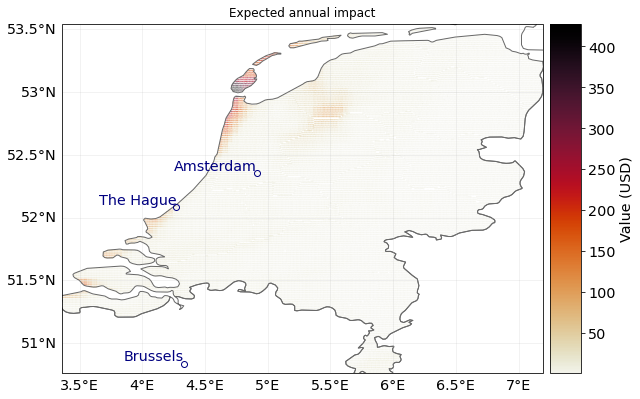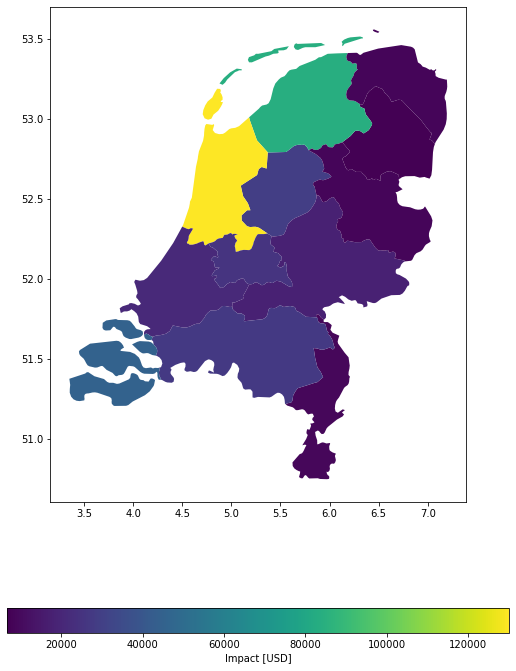Aggregated impact, in detail

# aggregate impact matrix
mat_agg = u_lp._aggregate_impact_mat(imp_pnt, exp_pnt3.gdf, agg_met=u_lp.AggMethod.SUM)

eai_exp = u_lp.eai_exp_from_mat(imp_mat=mat_agg, freq=imp_pnt.frequency)
at_event = u_lp.at_event_from_mat(imp_mat=mat_agg)
aai_agg = u_lp.aai_agg_from_at_event(at_event=at_event, freq=imp_pnt.frequency)

eai_exp

array([  6753.45186608,  28953.21638482,  84171.28903387,  18014.15630989,
8561.18507994,   8986.13653385,  27446.46387061, 130145.29903078,
8362.17243334,  20822.87844894,  25495.46296087,  45121.14833362])

at_event

array([4321211.03400214,  219950.4291506 ])

aai_agg

412832.8602866131


## Lines#

Lines are common geographical representation of transport infrastructure like streets, train tracks or powerlines etc. Here we will play it through for the case of winter storm Lothar’s impact on the Dutch Railway System:

Note: Hazard and impact functions data have been loaded above.

def gdf_lines():
gdf_lines = gdf_lines.to_crs(epsg=4326)
return gdf_lines

exp_nl_lines = Exposures(gdf_lines())
exp_nl_lines.gdf['impf_WS'] = 1
exp_nl_lines.gdf['value'] = 1

distance geometry impf_WS value
0 9414.978692 LINESTRING (6.08850 50.87940, 6.08960 50.87850... 1 1
1 10397.965112 LINESTRING (6.17673 51.35530, 6.17577 51.35410... 1 1
2 26350.219724 LINESTRING (5.68096 51.25040, 5.68422 51.25020... 1 1
3 40665.249638 LINESTRING (5.68096 51.25040, 5.67711 51.25030... 1 1
4 8297.689753 LINESTRING (5.70374 50.85490, 5.70531 50.84990... 1 1
exp_nl_lines.gdf.plot('value', cmap='inferno');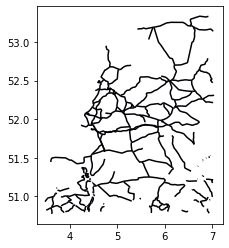### Calculating line impacts - all in one#

Example 1: Disaggregate values evenly among road segments; split in points with 0.005 degree distances.

imp_deg = u_lp.calc_geom_impact(
exp=exp_nl_lines, impf_set=impf_set, haz=storms,
res=0.005, disagg_met=u_lp.DisaggMethod.DIV, disagg_val=None,
agg_met=u_lp.AggMethod.SUM
)

/Users/ckropf/Documents/Climada/climada_python/climada/util/lines_polys_handler.py:931: UserWarning: Geometry is in a geographic CRS. Results from 'length' are likely incorrect. Use 'GeoSeries.to_crs()' to re-project geometries to a projected CRS before this operation.

line_lengths = gdf_lines.length

2022-06-24 13:16:59,608 - climada.entity.exposures.base - INFO - Setting latitude and longitude attributes.
2022-06-24 13:16:59,787 - climada.entity.exposures.base - INFO - Matching 10175 exposures with 9944 centroids.
2022-06-24 13:16:59,789 - climada.util.coordinates - INFO - No exact centroid match found. Reprojecting coordinates to nearest neighbor closer than the threshold = 100
2022-06-24 13:16:59,805 - climada.engine.impact - INFO - Exposures matching centroids found in centr_WS
2022-06-24 13:16:59,806 - climada.engine.impact - INFO - Calculating damage for 10175 assets (>0) and 2 events.

u_lp.plot_eai_exp_geom(imp_deg);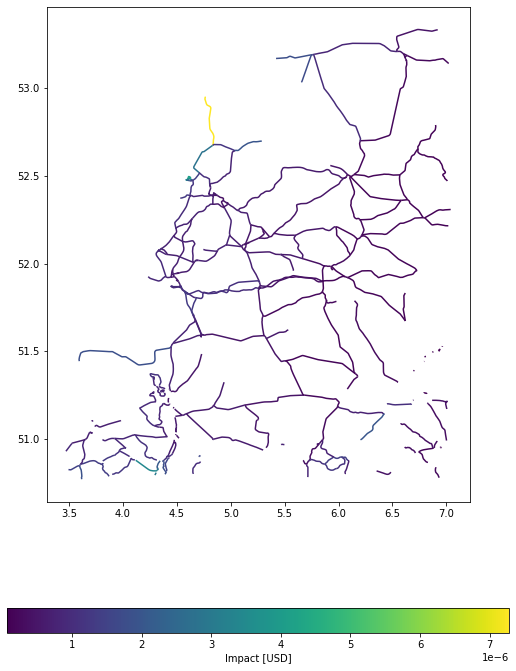Example 2: Disaggregate values evenly among road segments; split in points with 500 m distances.

imp_m = u_lp.calc_geom_impact(
exp=exp_nl_lines, impf_set=impf_set, haz=storms,
res=500, to_meters=True, disagg_met=u_lp.DisaggMethod.DIV, disagg_val=None,
agg_met=u_lp.AggMethod.SUM
)

2022-06-24 13:17:00,670 - climada.entity.exposures.base - INFO - Setting latitude and longitude attributes.
2022-06-24 13:17:00,827 - climada.entity.exposures.base - INFO - Matching 8399 exposures with 9944 centroids.
2022-06-24 13:17:00,828 - climada.util.coordinates - INFO - No exact centroid match found. Reprojecting coordinates to nearest neighbor closer than the threshold = 100
2022-06-24 13:17:00,843 - climada.engine.impact - INFO - Exposures matching centroids found in centr_WS
2022-06-24 13:17:00,845 - climada.engine.impact - INFO - Calculating damage for 8399 assets (>0) and 2 events.

u_lp.plot_eai_exp_geom(imp_m);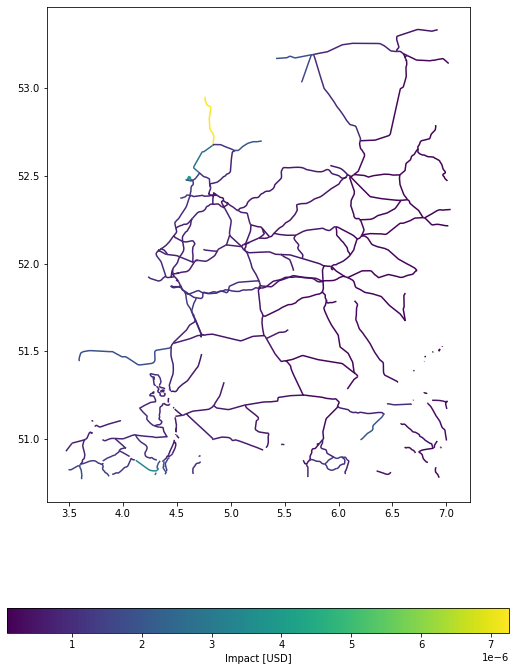import numpy as np
diff = np.max((imp_deg.eai_exp - imp_m.eai_exp) / imp_deg.eai_exp)
print(f"The largest relative different between degrees and meters impact in this example is {diff}")

The largest relative different between degrees and meters impact in this example is 0.09803913811822067


### Calculating line impacts - step by step#

Step 1: As in the polygon example above, there are several methods to disaggregate line exposures into point exposures, of which several are shown here:

# 0.1° distance between points, average value disaggregation
exp_pnt = u_lp.exp_geom_to_pnt(
exp_nl_lines, res=0.1, to_meters=False, disagg_met=u_lp.DisaggMethod.DIV, disagg_val=None
)

2022-06-24 13:17:01,409 - climada.entity.exposures.base - INFO - Setting latitude and longitude attributes.

/Users/ckropf/Documents/Climada/climada_python/climada/util/lines_polys_handler.py:931: UserWarning: Geometry is in a geographic CRS. Results from 'length' are likely incorrect. Use 'GeoSeries.to_crs()' to re-project geometries to a projected CRS before this operation.

line_lengths = gdf_lines.length

distance geometry_orig impf_WS value geometry latitude longitude
0 0 9414.978692 LINESTRING (6.08850 50.87940, 6.08960 50.87850... 1 0.500000 POINT (6.08850 50.87940) 50.879400 6.088500
1 9414.978692 LINESTRING (6.08850 50.87940, 6.08960 50.87850... 1 0.500000 POINT (6.06079 50.80030) 50.800300 6.060790
1 0 10397.965112 LINESTRING (6.17673 51.35530, 6.17577 51.35410... 1 0.333333 POINT (6.17673 51.35530) 51.355300 6.176730
1 10397.965112 LINESTRING (6.17673 51.35530, 6.17577 51.35410... 1 0.333333 POINT (6.12632 51.32440) 51.324399 6.126323
2 10397.965112 LINESTRING (6.17673 51.35530, 6.17577 51.35410... 1 0.333333 POINT (6.08167 51.28460) 51.284600 6.081670
# 1000m distance between points, no value disaggregation
exp_pnt2 = u_lp.exp_geom_to_pnt(
exp_nl_lines, res=1000, to_meters=True, disagg_met=u_lp.DisaggMethod.FIX, disagg_val=None
)

2022-06-24 13:17:01,876 - climada.entity.exposures.base - INFO - Setting latitude and longitude attributes.

distance geometry_orig impf_WS value geometry latitude longitude
0 0 9414.978692 LINESTRING (6.08850 50.87940, 6.08960 50.87850... 1 1 POINT (6.08850 50.87940) 50.879400 6.088500
1 9414.978692 LINESTRING (6.08850 50.87940, 6.08960 50.87850... 1 1 POINT (6.09416 50.87275) 50.872755 6.094165
2 9414.978692 LINESTRING (6.08850 50.87940, 6.08960 50.87850... 1 1 POINT (6.09161 50.86410) 50.864105 6.091608
3 9414.978692 LINESTRING (6.08850 50.87940, 6.08960 50.87850... 1 1 POINT (6.08744 50.85590) 50.855902 6.087435
4 9414.978692 LINESTRING (6.08850 50.87940, 6.08960 50.87850... 1 1 POINT (6.08326 50.84770) 50.847699 6.083263
# 1000m distance between points, equal value disaggregation
exp_pnt3 = u_lp.exp_geom_to_pnt(
exp_nl_lines, res=1000, to_meters=True, disagg_met=u_lp.DisaggMethod.DIV, disagg_val=None
)

2022-06-24 13:17:02,436 - climada.entity.exposures.base - INFO - Setting latitude and longitude attributes.

distance geometry_orig impf_WS value geometry latitude longitude
0 0 9414.978692 LINESTRING (6.08850 50.87940, 6.08960 50.87850... 1 0.090909 POINT (6.08850 50.87940) 50.879400 6.088500
1 9414.978692 LINESTRING (6.08850 50.87940, 6.08960 50.87850... 1 0.090909 POINT (6.09416 50.87275) 50.872755 6.094165
2 9414.978692 LINESTRING (6.08850 50.87940, 6.08960 50.87850... 1 0.090909 POINT (6.09161 50.86410) 50.864105 6.091608
3 9414.978692 LINESTRING (6.08850 50.87940, 6.08960 50.87850... 1 0.090909 POINT (6.08744 50.85590) 50.855902 6.087435
4 9414.978692 LINESTRING (6.08850 50.87940, 6.08960 50.87850... 1 0.090909 POINT (6.08326 50.84770) 50.847699 6.083263
# 1000m distance between points, disaggregation of value according to representative distance
exp_pnt4 = u_lp.exp_geom_to_pnt(
exp_nl_lines, res=1000, to_meters=True,  disagg_met=u_lp.DisaggMethod.FIX, disagg_val=1000
)

2022-06-24 13:17:03,116 - climada.entity.exposures.base - INFO - Setting latitude and longitude attributes.